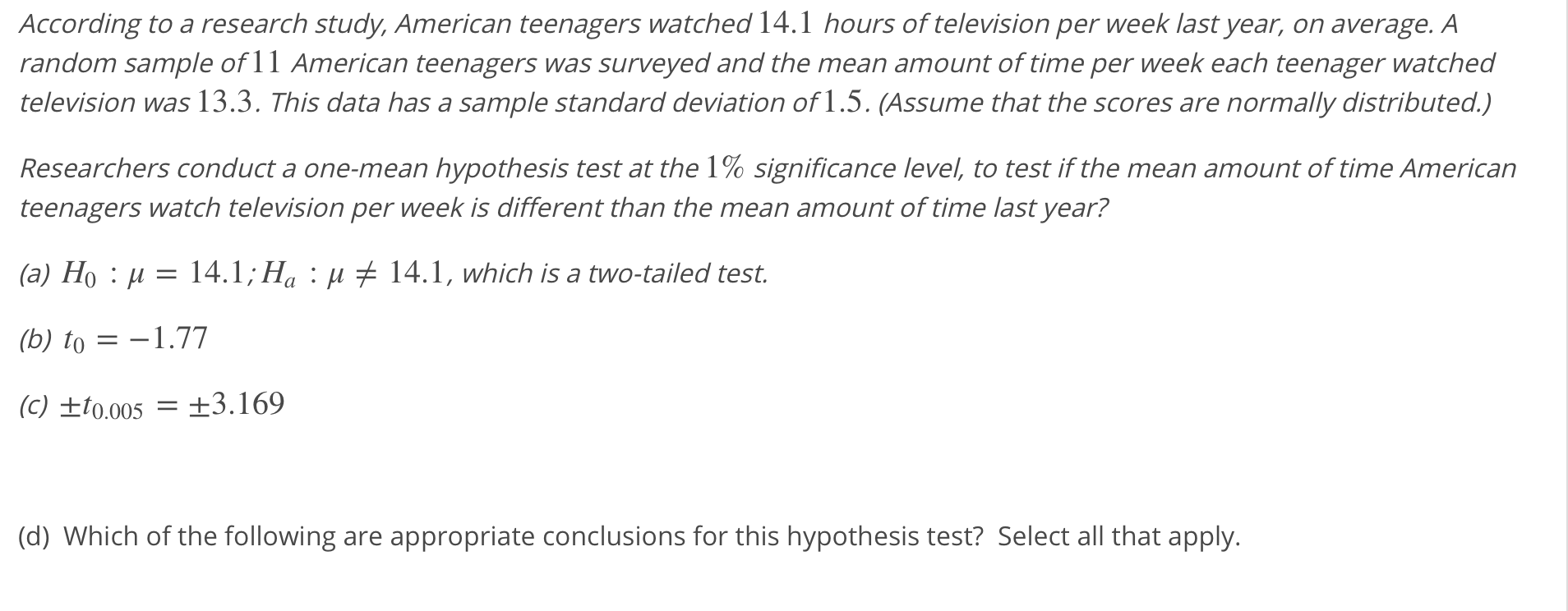# According to a research study,American teenagers watched 14.1 hours of television per week last year, on average. Arandom sample of 11 American teenagers was surveyed and the mean amount of time per week each teenager watchedtelevision was 13.3. This data has a sample standard deviation of 1.5. (Assume that the scores are normally distributed.)Researchers conduct a one-mean hypothesis test at the 1% significance level, to test if the mean amount of time Americanteenagers watch television per week is different than the mean amount of time last year?(a) Ho : μ-14.1: Ha : μ(b) to-1.77(C) +10005 3.16914.1, which is a two-tailed test.(d) Which of the following are appropriate conclusions for this hypothesis test? Select all that apply.

Question

Select all that apply:

•

We should reject H0.

We should not reject H0.

•

At the 1% significance level, the data do not provide sufficient evidence to conclude that the amount of time American teenagers watch television per week is different from 14.1 hours.

•

At the 1% significance level, the data provide sufficient evidence to conclude that the amount of time American teenagers watch television per week is different from 14.1 hours.help_outlineImage TranscriptioncloseAccording to a research study,American teenagers watched 14.1 hours of television per week last year, on average. A random sample of 11 American teenagers was surveyed and the mean amount of time per week each teenager watched television was 13.3. This data has a sample standard deviation of 1.5. (Assume that the scores are normally distributed.) Researchers conduct a one-mean hypothesis test at the 1% significance level, to test if the mean amount of time American teenagers watch television per week is different than the mean amount of time last year? (a) Ho : μ-14.1: Ha : μ (b) to-1.77 (C) +10005 3.169 14.1, which is a two-tailed test. (d) Which of the following are appropriate conclusions for this hypothesis test? Select all that apply. fullscreen
check_circleExpert Solution
Step 1

Test hypothesis:

Denote the population mean amount of time American teenagers watch television per week as μ.

The hypotheses are given below:

Null hypothesis:

H0 : μ = 14.1 hours

That is, the mean amount of time American teenagers watch television per week is equal to 14.1 hours.

Alternative hypothesis:

Ha : μ ≠ 14.1 hours (Two tail test).

That is, the mean amount of time American teenagers watch television per week is not equal to 14.1 hours.

Step 2

Given information:

The level of significance is 1%.

The test statistic value of the hypothesis test is t0 = –1.77.

The critical value for the hypothesis test is t(α/2) = ± 3.169.

Step 3

Decision rule:

Denote t0 as test statistic value and t(α/2) as the critical value.

Decision rule based on critical approach:

If t0 ≤ –t(α/2) (or) t0 ≥ t(α/2), then reject the null hypothesis H0.

If –t(α/2) < t0...

### Want to see the full answer?

See Solution

#### Want to see this answer and more?

Solutions are written by subject experts who are available 24/7. Questions are typically answered within 1 hour*

See Solution
*Response times may vary by subject and question
Tagged in

### Other